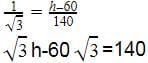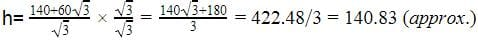# Test: Applications of Heights & Distances

## 10 Questions MCQ Test NCERT Mathematics for CAT Preparation | Test: Applications of Heights & Distances

Description
Attempt Test: Applications of Heights & Distances | 10 questions in 10 minutes | Mock test for Class 10 preparation | Free important questions MCQ to study NCERT Mathematics for CAT Preparation for Class 10 Exam | Download free PDF with solutions
QUESTION: 1

Solution:
QUESTION: 2

Solution:
QUESTION: 3

### A vertical tower is 20 m high. A man at some distance from the tower knows that the cosine of the angle of the elevation of the top of tower is 0.5. He is standing from the foot of the tower at a distance of:

Solution:
QUESTION: 4

A tower stands vertically on the ground. From a point on the ground 30 m away from the foot of the tower, the angle of elevation of the top of the tower is 45o. The height of the tower will be

Solution:
QUESTION: 5

The horizontal distance between two towers is 140 m. The angles of depression of the first tower, when seen from the top of the second tower is 30°. If the height of the first tower is 60 m. Find the height of the second tower.

Solution:

The second tower is smaller than the first tower so let the height of the first tower is h So a part of it is equal to 60m so the remaining height is h-60 m
Tan 30=perpendicular/baseQUESTION: 6

A man is standing on the deck of a ship, which is 8 m above water level. He observes the angle of elevation of the top of a hill as 60° and angle of depression of the base of the hill as 30°. What is the height of the hill?

Solution:
QUESTION: 7

An observer 1.5 m tall is 28.5 m away from a tower. The angle of elevation of the top of the tower from his eyes is 45°. The height of the tower is​

Solution:
QUESTION: 8

The shadow of a flag pole is 30 metres. If the altitude of the sun is at 30° then the height of the flag pole is

Solution:
QUESTION: 9

If the angle of elevation of a cloud from a point 100 metres above a lake is 30o and the angle of depression of its reflection in the lake is 60°, then the height of the cloud above the lake is​

Solution:
QUESTION: 10

A tree is broken by wind and its upper part touches the ground at a point 10 metres from the foot of the tree and makes an angle of 45° with the ground. The entire length of the tree is​

Solution:Use Code STAYHOME200 and get INR 200 additional OFF Use Coupon Code